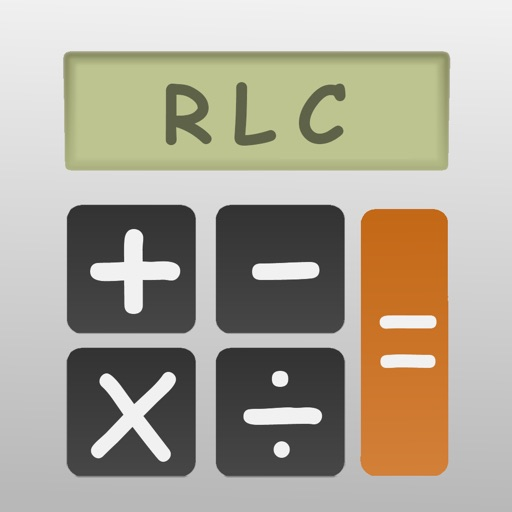EE Calculator# EE Calculator

Free
0

0 Ratings

Update Date

2020-02-21

Size

7.1 MB

### Screenshots for iPhone

iPhone
EE Calculator Description
This app is a collection of basic electronic calculators. It is suitable for hobbyist, electronic engineers or professionals.

Features
• Calculate resistance of 2 resistors in series / parallel
• To find out combinations of 2 resistors in series / in parallel making a desired resistance
• Calculate ratio of voltage divider and find out combinations of resistor making a desired ratio
• Calculate inverting amplifier gain and find out combinations of resistor making a desired ratio
• Ohm's Law calculation
• Star-delta conversion
• RC charging circuit : Calculate time constant by R and C
• RC Filter : Calculate cutoff frequency by resistor and capacitor value and find out combinations of resistance and capacitance making a desired cutoff frequency
• RL Filter : Calculate cutoff frequency by resistor and inductor value and find out combinations of resistance and inductance making a desired cutoff frequency
• LC Filter : Calculate resonant frequency by capacitor and inductor values and find out combinations of capacitance and inductance making a desired resonant frequency
• Calculate monostable circuit
• Calculate frequency of astable circuit and find out combinations of capacitor and resistor making a desired astable frequency
• Save combinations in CSV file
* Upload generated CSV files via AirDrop or iCloud Drive
EE Calculator 2.9.6 Update
2020-02-21 Version History
Fix minor bugs
More
Price:
Free
Version:
2.9.6
Size:
7.1 MB
Genre:
Utilities
Update Date:
2020-02-21
Developer:
Siu Yuen Ho
Language:
English
More
You May Also Like
Developer Apps